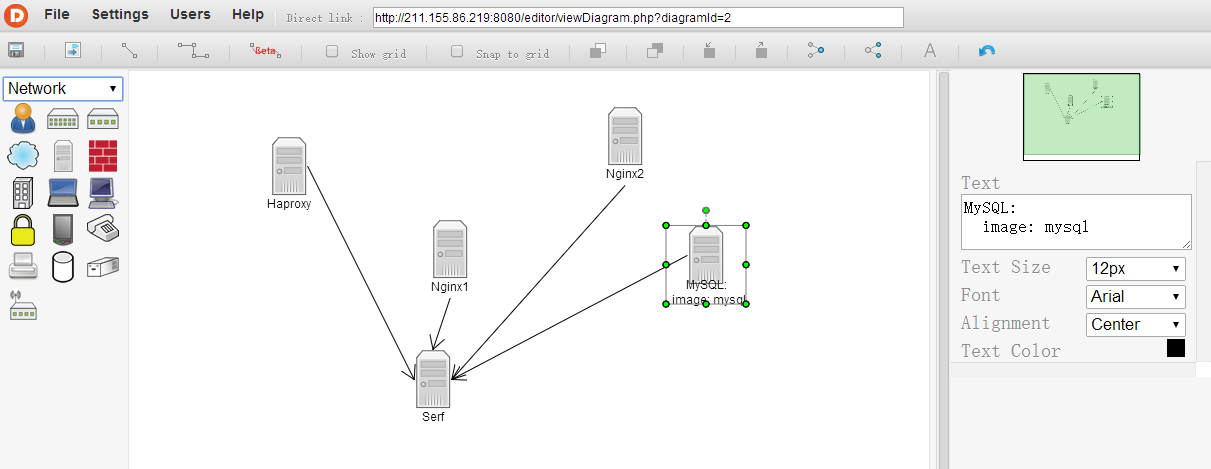Apache/Nginx 上配置的 `server_name` 必须跟你浏览器访问的完全一致

(写到这里感慨一下，chrome的调试工具不会用，这问题最后还是在 IE开发者工具的帮助下发现的 ==！)``````use File::Slurp;
use JSON;
use YAML;
use Test::Deep::NoTest;
use 5.010;
use warnings;
use strict;
my \$hash = from_json( read_file( \$ARGV ) );
my \$hostinfo;
for my \$host ( @{ \$hash->{s}->{figures} } ) {
\$hostinfo->{ \$host->{id} } = Load( \$host->{primitives}->->{str} );
}
for my \$conn ( @{ \$hash->{m}->{connectors} } ) {
my \$connid = \$conn->{id};
my \$start  = \$conn->{turningPoints}->;
my \$end    = \$conn->{turningPoints}->;
if ( \$conn->{endStyle} eq 'Normal' and \$conn->{startStyle} eq 'Arrow' ) {
( \$start, \$end ) = ( \$end, \$start );
}
my ( \$startid, \$endid );
for my \$point ( @{ \$hash->{m}->{connectionPoints} } ) {
if (    eq_deeply( \$point->{point}, \$start )
and \$point->{parentId} != \$connid
and exists \$hostinfo->{ \$point->{parentId} } )
{
\$startid = \$point->{parentId};
}
elsif ( eq_deeply( \$point->{point}, \$end )
and \$point->{parentId} != \$connid
and exists \$hostinfo->{ \$point->{parentId} } )
{
\$endid = \$point->{parentId};
}
}
my (\$startname) = keys %{ \$hostinfo->{\$startid} };
my (\$endname) = keys %{ \$hostinfo->{\$endid} };
}
say Dump { map { my (\$k) = keys \$_; \$k => \$_->{\$y} } values \$hostinfo};
``````

``````---
Haproxy:
- Serf
Nginx1: